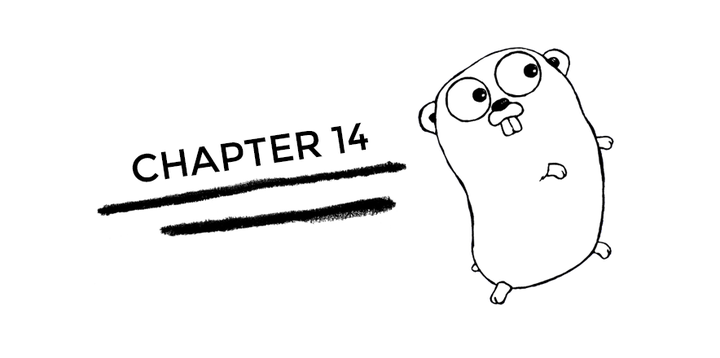# Golang - Chapter 14

InterfacesGo does not explicitly say that a struct implements an interface. If a struct implements all the methods of an interface, it automatically becomes of the interface type. Thus, in Go, interfaces are implemented implicitly.

Therefore, in Go, an interface type is a set of method signatures. The best way to understand interfaces is to see it in action.

``````package main

import "fmt"

type Shape interface {
area() float64
perimeter() float64
}

// This is a right-angled triangle
type Triangle struct {
base, height, hypotenuse float64
}

type Square struct {
length float64
}

// Triangle struct implicitly implements the `shape` interfce
func (tri Triangle) area() float64 {
return 0.5 * tri.base * tri.height
}

func (tri Triangle) perimeter() float64 {
return tri.base + tri.height + tri.hypotenuse
}

// Square struct implicitly implements the `shape` interfce
func (sq Square) area() float64 {
return sq.length * sq.length
}

func (sq Square) perimeter() float64 {
return sq.length * 4.0
}

// Now this method would work for both Triangle and Square
func printProperties(s Shape) {
fmt.Println(s.area())
fmt.Println(s.perimeter())
fmt.Println()
}

func main() {
s := Square{length: 5}
t := Triangle{base: 3, height: 4, hypotenuse: 5}

// we can call printProperties on both s and t
printProperties(s)
printProperties(t)
}``````

In the snippet above, we have defined `Shape` to be an interface that provides the methods `area()` and `perimeter()`. Any struct that implements these two methods will become a `Shape`.

We then define two structs - `Square` and `Triangle` and implement the methods `area()` and `perimeter()` for each of them, allowing them to be a `Shape`.

We then define a function called `printProperties()` which prints the area and perimeter of a `Shape` provided as argument.

As you would see if you run this program, the method `printProperties()` works on both `s` and `t` which are of type `Square` and `Triangle` respectively.

Side note: The above example actually depicts polymorphism - ability for instances to behave as other types. Check out more on polymorphism here.I code.